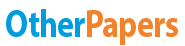# Density of Pennies

Essay by   •  October 10, 2012  •  Study Guide  •  1,020 Words (5 Pages)  •  1,389 Views

## Essay Preview: Density of Pennies

Report this essay
Page 1 of 5

Density of Pennies

Introduction/Purpose:

The purpose of this laboratory experiment was to learn how to properly calculate density. Also another purpose was to learn how to correctly use water displacement to calculate the density of pennies.

Materials:

* 15 pennies minted before 1982

* 15 pennies minted in or after 1982

* Triple Beam Balance

Procedure:

1. Check to see if your balance pointer is on zero while all the movable masses are on zero. This will get you more accurate results.

2. Place your weigh paper on the pan.

3. Then move the movable masses until your pointer evens out at zero again.

4. Record the mass of the weigh paper.

6. While keeping the weigh paper on the pan, determine the mass of 15 pennies minted before 1982. Repeat your measurement two more times, for a total of 3 trials.

7. Repeat all of step 6 with the 15 pennies minted in 1982 or after.

8. Pour 25 mL of water into the 50 mL graduated cylinder. Add the 15 pennies minted before 1982 into the graduated cylinder. Find and record the volume of the pennies

9. Repeat step 8 with the pennies minted in 1982 or after.

10. Review your data for any large differences between the trials. If there are any large differences, repeat the appropriate measurement.

Quantitative Data:

Mass of Pennies

Object Trial 1 (grams) Trial 2 (grams) Trial 3 (grams)

Weigh Paper 0.90 0.90 0.90

Pennies made before 1982 47.20 47.20 47.20

Pennies made in 1982 or later 38.50 38.55 38.53

Volume of Pennies

Volume mL

Water 25.0

Water w/ pennies made before 1982 30.8

Water 25.0

Water w/ pennies made in 1982 or later 30.9

Calculations:

(Trial 1) + (Trial 2) + (Trial 3) divided by 3 subtract (Weigh Paper):

47.20 g + 47.20 g + 47.20 g = 141.6 g

141.6 g divided by 3 = 47.20 g

47.20 g subtracted by .90 g = 46.30 g

The average mass of the 15 pennies minted before 1982 is 46.30 grams.

Pennies made in 1982 or later:

(Trial 1) + (Trial 2) + (Trial 3) divided by 3 subtract (Weigh Paper):

38.50 g + 38.55 g + 38.53 g = 115.58 g

115.58 g divided by 3 = 38.52 g

38.52 g subtracted by .90 g = 37.62 g

The average mass of the pennies minted in 1982 or later is 37.62 grams.

(Water/Pennies made before 1982) subtracted by (Water):

30.8 mL subtracted by 25.0 mL = 5.80 mL

The volume of the pennies made before 1982 is 5.80 mL.

Pennies made in 1982 or later:

(Water/ Pennies made in 1982 or later subtracted by (Water):

30.9 mL subtracted by 25.0 mL = 5.90 mL

The volume of the pennies minted in 1982 or later is 5.90 mL.

(Average mass of the pennies minted before 1982) divided by 15 (# of pennies)

46.30

...

...